Lugano tutorial: Metadynamics simulations with PLUMED

# Aims

The aim of this tutorial is to train users to perform metadynamics simulations with PLUMED. Error analysis, which is very very important and requires extensive discussions, will be done in a separate tutorial.

# Objectives

Once this tutorial is completed students will be able to:

• Write the PLUMED input file to perform metadynamics simulations
• Calculate the free energy from a metadynamics run
• Monitor the behavior of variables in a metadynamics run

# Resources

The for this project contains the following files:

• diala.pdb: a PDB file for alanine dipeptide in vacuo
• topol.tpr: a GROMACS run file to perform MD of alanine dipeptide

This tutorial has been tested on version 2.5.

# Introduction

We have seen that PLUMED can be used to compute collective variables. However, PLUMED is most often use to add forces on the collective variables. To this aim, we have implemented a variety of possible biases acting on collective variables. The complete documentation for all the biasing methods available in PLUMED can be found at the Bias page. In the following we will see how to build an adaptive bias potential with metadynamics. Here you can find a brief recap of the metadynamics theory.

We will play with a toy system, alanine dipeptide simulated in vacuo using the AMBER99SB-ILDN force field (see Fig. lugano-3-ala-fig). This rather simple molecule is useful to understand data analysis and free-energy methods. This system is a nice example because it presents two metastable states separated by a high free-energy barrier. It is conventional use to characterize the two states in terms of Ramachandran dihedral angles, which are denoted with $$\Phi$$ and $$\Psi$$ in Fig. lugano-3-transition-fig .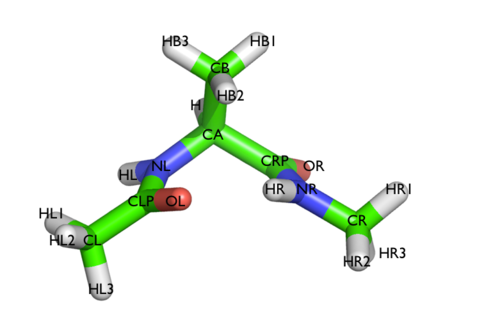The molecule of the day: alanine dipeptide.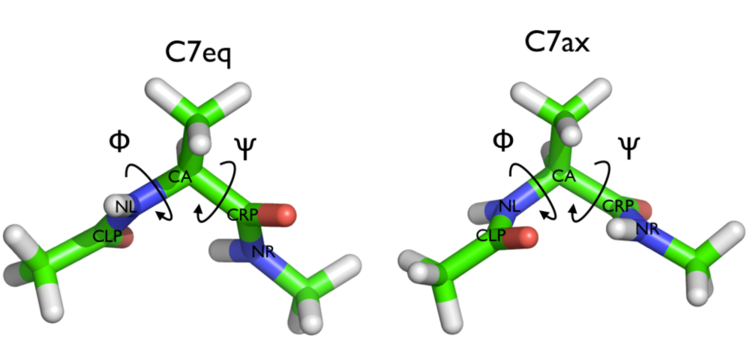Two metastable states of alanine dipeptide are characterized by their Ramachandran dihedral angles.

## Exercise 1: my first metadynamics calculation

In this exercise we will setup and perform a well-tempered metadynamics run using the backbone dihedral $$\phi$$ as collective variable. During the calculation, we will also monitor the behavior of the other backbone dihedral $$\psi$$.

Here you can find a sample plumed.dat file that you can use as a template. Whenever you see an highlighted FILL string, this is a string that you should replace.

Click on the labels of the actions for more information on what each action computes# vim:ft=plumed
MOLINFO STRUCTUREcompulsory keyword
a file in pdb format containing a reference structure. =diala.pdb
# Compute the backbone dihedral angle phi, defined by atoms C-N-CA-C
# you might want to use MOLINFO shortcuts
phi: TORSION ATOMSthe four atoms involved in the torsional angle =__FILL__
# Compute the backbone dihedral angle psi, defined by atoms N-CA-C-N
psi: TORSION ATOMSthe four atoms involved in the torsional angle =__FILL__
# Activate well-tempered metadynamics in phi
metad: __FILL__ ARG could not find this keyword =__FILL__
# Deposit a Gaussian every 500 time steps, with initial height equal to 1.2 kJ/mol
PACE could not find this keyword =500 HEIGHT could not find this keyword =1.2
# the bias factor should be wisely chosen
BIASFACTOR could not find this keyword =__FILL__
# Gaussian width (sigma) should be chosen based on CV fluctuation in unbiased run
SIGMA could not find this keyword =__FILL__
# Gaussians will be written to file and also stored on grid
FILE could not find this keyword =HILLS GRID_MIN could not find this keyword =-pi GRID_MAX could not find this keyword =pi
...
# Print both collective variables and the value of the bias potential on COLVAR file
PRINT ARGthe input for this action is the scalar output from one or more other actions. =__FILL__ FILEthe name of the file on which to output these quantities =COLVAR STRIDEcompulsory keyword ( default=1 )
the frequency with which the quantities of interest should be output =10


The syntax for the command METAD is simple. The directive is followed by a keyword ARG followed by the labels of the CVs on which the metadynamics potential will act. The keyword PACE determines the stride of Gaussian deposition in number of time steps, while the keyword HEIGHT specifies the height of the Gaussian in kJ/mol. For each CVs, one has to specify the width of the Gaussian by using the keyword SIGMA. Gaussian will be written to the file indicated by the keyword FILE.

In this example, the bias potential will be stored on a grid, whose boundaries are specified by the keywords GRID_MIN and GRID_MAX. Notice that you can provide either the number of bins for every collective variable (GRID_BIN) or the desired grid spacing (GRID_SPACING). In case you provide both PLUMED will use the most conservative choice (highest number of bins) for each dimension. In case you do not provide any information about bin size (neither GRID_BIN nor GRID_SPACING) and if Gaussian width is fixed, PLUMED will use 1/5 of the Gaussian width as grid spacing. This default choice should be reasonable for most applications.

Once your plumed.dat file is complete, you can run a 10-ns long metadynamics simulations with the following command

> gmx mdrun -s topol.tpr -nsteps 5000000 -plumed plumed.dat


During the metadynamics simulation, PLUMED will create two files, named COLVAR and HILLS. The COLVAR file contains all the information specified by the PRINT command, in this case the value of the CVs every 10 steps of simulation, along with the current value of the metadynamics bias potential. We can use gnuplot to visualize the behavior of the CV during the simulation, as reported in the COLVAR file:

gnuplot> p "COLVAR" u 1:2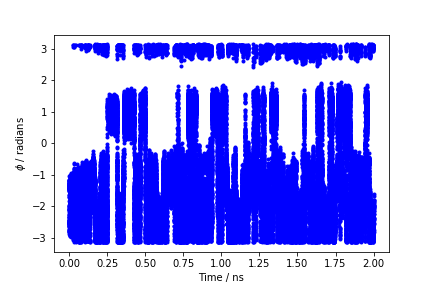Time evolution of the metadynamics CV during the first 2 ns of a metadynamics simulation of alanine dipeptide in vacuum.

By inspecting Figure lugano-3-phi-fig, we can see that the system is initialized in one of the two metastable states of alanine dipeptide. After a while (t=0.1 ns), the system is pushed by the metadynamics bias potential to visit the other local minimum. As the simulation continues, the bias potential fills the underlying free-energy landscape, and the system is able to diffuse in the entire phase space.

The HILLS file contains a list of the Gaussian kernels deposited along the simulation. If we give a look at the header of this file, we can find relevant information about its content:

#! FIELDS time phi sigma_phi height biasf
#! SET multivariate false
#! SET min_phi -pi
#! SET max_phi pi


The line starting with FIELDS tells us what is displayed in the various columns of the HILLS file: the simulation time, the instantaneous value of $$\phi$$, the Gaussian width and height, and the bias factor. We can use the HILLS file to visualize the decrease of the Gaussian height during the simulation, according to the well-tempered recipe: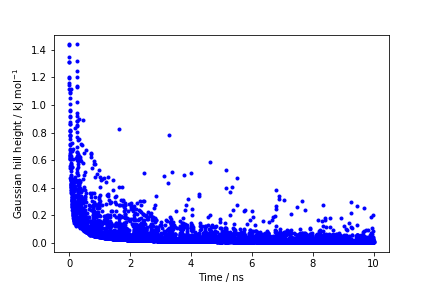Time evolution of the Gaussian height.

If we look carefully at the scale of the y-axis, we will notice that in the beginning the value of the Gaussian height is higher than the initial height specified in the input file, which should be 1.2 kJ/mol. In fact, this column reports the height of the Gaussian scaled by the pre-factor that in well-tempered metadynamics relates the bias potential to the free energy.

## Exercise 2: estimating the free energy

One can estimate the free energy as a function of the metadynamics CVs directly from the metadynamics bias potential. In order to do so, the utility sum_hills should be used to sum the Gaussian kernels deposited during the simulation and stored in the HILLS file.
To calculate the free energy as a function of $$\phi$$, it is sufficient to use the following command line:

plumed sum_hills --hills HILLS


The command above generates a file called fes.dat in which the free-energy surface as function of $$\phi$$ is calculated on a regular grid. One can modify the default name for the free energy file, as well as the boundaries and bin size of the grid, by using the following options of sum_hills :

--outfile - specify the outputfile for sumhills
--min - the lower bounds for the grid
--max - the upper bounds for the grid
--bin - the number of bins for the grid
--spacing - grid spacing, alternative to the number of bins


The result should look like this: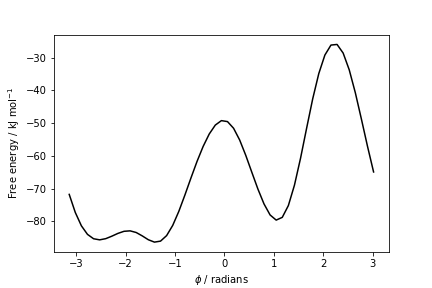Estimate of the free energy as a function of the dihedral phi from a 10ns-long well-tempered metadynamics simulation.

To assess the convergence of a metadynamics simulation, one can calculate the estimate of the free energy as a function of simulation time. At convergence, the reconstructed profiles should be similar. The option --stride should be used to give an estimate of the free energy every N Gaussian kernels deposited, and the option --mintozero can be used to align the profiles by setting the global minimum to zero. If we use the following command line:

plumed sum_hills --hills HILLS --stride 100 --mintozero


one free energy is calculated every 100 Gaussian kernels deposited, and the global minimum is set to zero in all profiles. The resulting plot should look like the following: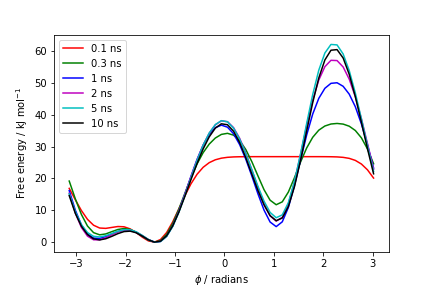Estimates of the free energy as a function of the dihedral phi calculated every 100 Gaussian kernels deposited.

These two qualitative observations:

• the system is diffusing efficiently in the collective variable space (Figure lugano-3-phi-fig)
• the estimated free energy does not change significantly as a function of time (Figure lugano-3-metad-phifest-fig)

suggest that the simulation most likely converged.

Warning
The fact that the Gaussian height is decreasing to zero should not be used as a measure of convergence of your metadynamics simulation!
Note
The two observations above are necessary, but qualitative conditions for convergence. A quantitative assessment of convergence can be obtained by performing an error analysis in a later tutorial.

## Exercise 3: the role of the bias factor.

The bias factor allows you to choose how extensive your sampling of the CV space will be. If you choose it too large, you will explore a large reason of the CV space and your simulation will take more time to converge. If you choose it too small, you will not be able to cross the barriers you are interested in.

Try to run your simulation with different values of the bias factor. Going low to anything that is greater than 1 should give meaningful results, although if the chosen value is too low the system will only explore a limited portion of the space.

Which is the minimum value of the bias factor that allows you to see, in the simulated time, transitions between the two relevant minima?

# Exercise 4: reweighting

In the previous exercise we biased $$\phi$$ and compute the free energy as a function of the same variable. Many times you want to decide which variable you want to analyze after having performed a simulation. In order to do so you must reweight your simulation.

There are multiple ways to reweight a metadynamics simulations. In order to calculate these weights, we can use either of these two approaches:

1) Weights are calculated by considering the time-dependence of the metadynamics bias potential ;

2) Weights are calculated using the metadynamics bias potential obtained at the end of the simulation and assuming a constant bias during the entire course of the simulation .

In this exercise we will use the umbrella-sampling-like reweighting approach (Method 2). In order to compute the weights we will use the driver tool.

First of all, prepare a plumed_reweight.dat file that is identical to the one you used for running your simulation but add the keyword RESTART=YES to the METAD command. This will make this action behave as if PLUMED was restarting. It will thus read the already accumulated hills and continue adding more. In addition, set hills height to zero and the pace to a large number. This will actually avoid adding new hills (and even if they are added they will have zero height). Finally, change the PRINT statement so that you write every frame (STRIDE=1) and that, in addition to phi and psi, you also write metad.bias.

Click on the labels of the actions for more information on what each action computes__FILL__ # here goes the definitions of the CVs
# Activate well-tempered metadynamics in phi
metad: __FILL__ ARG could not find this keyword =__FILL__
# Deposit a Gaussian every 500 time steps, with initial height equal to 1.2 kJ/mol
PACE could not find this keyword =10000000 HEIGHT could not find this keyword =0.0 # <- this is the new stuff!
# the bias factor should be wisely chosen
BIASFACTOR could not find this keyword =__FILL__
# Gaussian width (sigma) should be chosen based on CV fluctuation in unbiased run
SIGMA could not find this keyword =__FILL__
# Gaussians will be written to file and also stored on grid
FILE could not find this keyword =HILLS GRID_MIN could not find this keyword =-pi GRID_MAX could not find this keyword =pi
# Say that METAD should be restarting
RESTART could not find this keyword =YES # <- this is the new stuff!
...
PRINT ARGthe input for this action is the scalar output from one or more other actions. =phi,psi,metad.bias FILEthe name of the file on which to output these quantities =COLVAR STRIDEcompulsory keyword ( default=1 )
the frequency with which the quantities of interest should be output =1 # <- also change this one!


Then run the driver using this command

> plumed driver --ixtc traj_comp.xtc --plumed plumed.dat --kt 2.5


Notice that you have to specify the value of $$k_BT$$ in energy units. While running your simulation this information was taken from the MD code.

As a result, PLUMED will produce a new COLVAR file with an additional column. The beginning of the file should look like this:

#! FIELDS time phi psi metad.bias
#! SET min_phi -pi
#! SET max_phi pi
#! SET min_psi -pi
#! SET max_psi pi
0.000000 -1.497988 0.273498 110.625670
1.000000 -1.449714 0.576594 110.873141
2.000000 -1.209587 0.831417 109.742353
3.000000 -1.475975 1.279726 110.752327


The last column will give as, in energy units, the logarithm of the weight of each frame. You can easily obtain the weight of each frame using the expression $$w\propto\exp\left(\frac{V(s)}{k_BT}\right)$$. You might want to read the COLVAR file in python and compute a weighted histogram.

If you want PLUMED to do the histograms for you, you can just add the following lines that you learned in Lugano tutorial: Using restraints to the plumed input file:

Click on the labels of the actions for more information on what each action computesas: REWEIGHT_BIAS ARGcompulsory keyword ( default=*.bias )
the biases that must be taken into account when reweighting =__FILL__
hhphi: HISTOGRAM ARGthe input for this action is the scalar output from one or more other actions. =phi STRIDEcompulsory keyword ( default=1 )
the frequency with which the data should be collected and added to the quantity being
averaged =50 GRID_MINcompulsory keyword
the lower bounds for the grid =-pi GRID_MAXcompulsory keyword
the upper bounds for the grid =pi GRID_BINthe number of bins for the grid =600 BANDWIDTHcompulsory keyword
the bandwidths for kernel density estimation =0.1 LOGWEIGHTSlist of actions that calculates log weights that should be used to weight configurations
when calculating averages =as
hhpsi: HISTOGRAM ARGthe input for this action is the scalar output from one or more other actions. =psi STRIDEcompulsory keyword ( default=1 )
the frequency with which the data should be collected and added to the quantity being
averaged =50 GRID_MINcompulsory keyword
the lower bounds for the grid =-pi GRID_MAXcompulsory keyword
the upper bounds for the grid =pi GRID_BINthe number of bins for the grid =600 BANDWIDTHcompulsory keyword
the bandwidths for kernel density estimation =0.1 LOGWEIGHTSlist of actions that calculates log weights that should be used to weight configurations
when calculating averages =as
ffphi: CONVERT_TO_FES GRIDcompulsory keyword
the action that creates the input grid you would like to use =hhphi
ffpsi: CONVERT_TO_FES GRIDcompulsory keyword
the action that creates the input grid you would like to use =hhpsi
DUMPGRID GRIDcompulsory keyword
the action that creates the grid you would like to output =ffphi FILEcompulsory keyword ( default=density )
the file on which to write the grid. =ffphi.dat
DUMPGRID GRIDcompulsory keyword
the action that creates the grid you would like to output =ffpsi FILEcompulsory keyword ( default=density )
the file on which to write the grid. =ffpsi.dat


and plot the result using gnuplot.

gnuplot> p "ffphi.dat"
gnuplot> p "ffpsi.dat"


# Exercise 5: larger orthogonal barriers

Alanine dipeptide is often considered as a too-simple system to understand the typical problems that one will then see in real systems. This is very much not true! We will here see how to make the exercise arbitrarily difficult, to the point that metadynamics will not work anymore.

The difficult case of metadynamics is when there are variables that (a) are orthogonal to the biased ones and (b) exhibit large energy barriers. The first point implies that when you flatten the distribution of the biased collective variables you are not accelerating the sampling of the orthogonal variables. The second point implies that those variable will take a lot of time to explore different metastable states.

Often these variables are not known and they are thus called "hidden variables". In the case of alanine dipeptide, we can easily add a barrier on $$\Psi$$ with some additional PLUMED command. We will add a Gaussian barrier centered at $$\Psi=0.5$$. To do so, add to the PLUMED input file that you prepared for the first exercise (that is: biasing $$\Phi$$ alone) the following lines

Click on the labels of the actions for more information on what each action computes# first shift the psi variable.
# setting the new periodicity to -pi,pi will make sure that the barrier
# is a continuous function of the coordinates
shift1: CUSTOM ARGthe input for this action is the scalar output from one or more other actions. =psi FUNCcompulsory keyword
the function you wish to evaluate =x-0.5 PERIODICcompulsory keyword
if the output of your function is periodic then you should specify the periodicity
of the function. =-pi,pi
shift2: CUSTOM ARGthe input for this action is the scalar output from one or more other actions. =psi FUNCcompulsory keyword
the function you wish to evaluate =x+2.5 PERIODICcompulsory keyword
if the output of your function is periodic then you should specify the periodicity
of the function. =-pi,pi
# then compute the barrier energy.
# this would be a Gaussian with wifth 0.3. You can pick the height as you like
barrier: CUSTOM ARGthe input for this action is the scalar output from one or more other actions. =shift1,shift2 FUNCcompulsory keyword
the function you wish to evaluate =__FILL__*exp(-0.5*x^2/0.2^2)+__FILL__*exp(-0.5*y^2/0.2^2) PERIODICcompulsory keyword
if the output of your function is periodic then you should specify the periodicity
of the function. =NO
# then add the barrier to the total energy of the system.
BIASVALUE ARGthe input for this action is the scalar output from one or more other actions. =barrier
`

Something high like 25 kj/mol should create a significant difficulty. Notice that this means a barrier 10 $$k_BT$$ high in a direction that is not being biased.

You should see something like this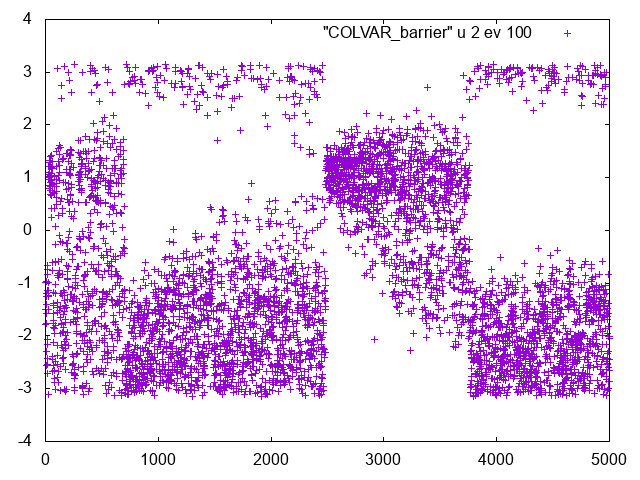Time series of Phi when Psi dynamics is hindered by a barrier

If you look at this series you will clearly see that there are clear changes in the Phi dynamics. If you look at Psi dynamics you should be able to see that these changes correspond to transitions in Psi.

This is an indication that an important slow variable is missing.

Find the minimum value of the barrier required for the simulation not to converge in the simulated timescale.

# Conclusions

In summary, in this tutorial you should have learned how to use PLUMED to:

• Setup and run a metadynamics calculation.
• Compute free energies from the metadynamics bias potential using the sum_hills utility.
• Identify problems when a hidden variable exhibit a large barrier